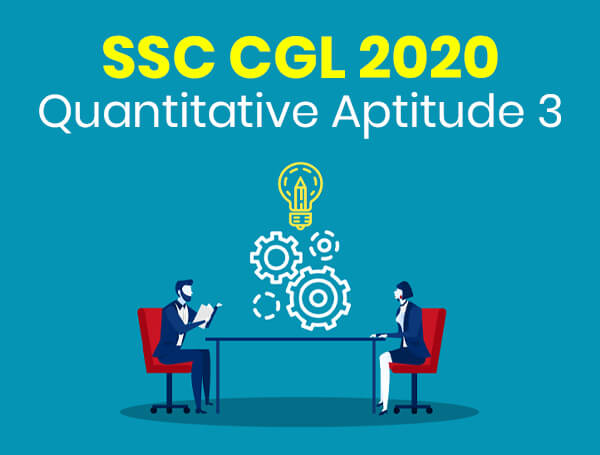SSC CGL 2020: Quantitative Aptitude 3 - Study24x7Default error msg

New to Study24x7 ?
By continuing, you agree to Study24x7 ?

# SSC CGL 2020: Quantitative Aptitude 3

Updated on 15 February 2020SSC Preparation Strategies & S
Updated on 15 February 2020The following topics of Quantitative Aptitude for the SSC CGL 2020 Exam are the most scoring.

Simplification: This is an important and one of the easy topics in quantitative aptitude. In simplification, you have to solve a complex calculation and to do this, you must learn the following-NOTE:- The Topics which this Article Cover is very important for SSC CGL 2020 Exam

1. BODMAS (Bracket of Division, multiplication, addition, and subtraction)

2. Memorize tables up to 25, or if you can, up to 30.

3. Instead of memorizing tricks to find out squares, square roots, cubes and cube roots, learn the trick to calculate them quickly. This will increase your speed and save time.

4. Fraction and its simplification

5. Surds and indices

Averages: In averages, you have to find the central value of a set of data. This is an important topic because the concept of averages is used in multiple questions. So mastering this topic will help you in quickly solving other questions too.

Averages can be further classified into the following-

1. Arithmetic Mean (AM) - In this, the sum of first and last terms are divided by 2 to get AM.

2. Geometric Mean (GM) - In this, the middle term of the series is GM. It can also be calculated in the following way-

GM of 2, 4 and 8 = (2x4x8)(1/3) = (64)(1/3) = 4

3. Harmonic Mean (HM) - HM is used in averaging values like rates. HM of two numbers, a and b will be 2/{(1/a)+(1/b)}.

Ratio and Proportion: Ratio is the comparison of two quantities of the same kind whereas proportion is the share of a quantity in the whole part. Just like averages, the concept of ratio and proportion can be used in multiple questions so make sure you learn the concepts thoroughly.

Interest: This is very important from the SSC CGL point of view and involves Simple Interest and Compound Interest.

Simple Interest - It is the interest calculated on money invested or borrowed at a certain rate for a certain amount of time.

SI = PxRxT/100

where P is the amount invested or borrowed; R is the rate of interest and T is the time.

Compound Interest - CI is the interest that gets accumulated over a period of time on the amount borrowed or invested initially.

CI = P{ [1+(R/100)]T} -1

Percentage: The concept of percentage is important for Data Interpretation and Data Analysis section too along with quantitative aptitude.

Percentage means per every hundred and is denoted by the "%" symbol. For example, if a student scores 20 marks out of 30, his percentage will be

(21/30) x100 = 70%

Another concept is the percentage change. When a quantity changes, the percentage change is calculated as

(Actual Change x Original Value) x 100

The preparation of SSC CGL 2020 exam requires speed and accuracy, and to achieve that you need to practice a lot. Also, remember that along with hard work you also need to do smart work. Identify the topics you are strong in and start with those. Practice till you feel confident about them and subsequently move to next topics.

All The Best To All The SSC CGL Aspirants !

Write a comment...Trending ArticlesBy CBSE CLASS 8By CBSE CLASS 10By CBSE CLASS 7By Edubabble LLP
Related Posts